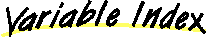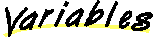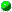`All Packages  Class Hierarchy  This Package  Previous  Next  Index`

# Class java.lang.Integer

```java.lang.Object
|
+----java.lang.Number
|
+----java.lang.Integer
```

public final class Integer
extends Number
The Integer class wraps a value of the primitive type `int` in an object. An object of type `Integer` contains a single field whose type is `int`.

In addition, this class provides several methods for converting an `int` to a `String` and a `String` to an `int`, as well as other constants and methods useful when dealing with an `int`.

##MAX_VALUE
The largest value of type `int`.MIN_VALUE
The smallest value of type `int`.TYPE
The Class object representing the primitive type int.

##Integer(int)
Constructs a newly allocated `Integer` object that represents the primitive `int` argument.Integer(String)
Constructs a newly allocated `Integer` object that represents the value represented by the string.

##byteValue()
Returns the value of this Integer as a byte.decode(String)
Decodes a string into an Integer.doubleValue()
Returns the value of this Integer as a double.equals(Object)
Compares this object to the specified object.floatValue()
Returns the value of this Integer as a float.getInteger(String)
Determines the integer value of the system property with the specified name.getInteger(String, int)
Determines the integer value of the system property with the specified name.getInteger(String, Integer)
Determines the integer value of the system property with the specified name.hashCode()
Returns a hashcode for this Integer.intValue()
Returns the value of this Integer as an int.longValue()
Returns the value of this Integer as a long.parseInt(String)
Parses the string argument as a signed decimal integer.parseInt(String, int)
Parses the string argument as a signed integer in the radix specified by the second argument.shortValue()
Returns the value of this Integer as a short.toBinaryString(int)
Creates a string representation of the integer argument as an unsigned integer in base 2.toHexString(int)
Creates a string representation of the integer argument as an unsigned integer in base 16.toOctalString(int)
Creates a string representation of the integer argument as an unsigned integer in base 8.toString()
Returns a String object representing this Integer's value.toString(int)
Returns a new String object representing the specified integer.toString(int, int)
Creates a string representation of the first argument in the radix specified by the second argument.valueOf(String)
Returns a new Integer object initialized to the value of the specified String.valueOf(String, int)
Returns a new Integer object initialized to the value of the specified String.

##MIN_VALUE
``` public static final int MIN_VALUE
```
The smallest value of type `int`.MAX_VALUE
``` public static final int MAX_VALUE
```
The largest value of type `int`.TYPE
``` public static final Class TYPE
```
The Class object representing the primitive type int.

##Integer
``` public Integer(int value)
```
Constructs a newly allocated `Integer` object that represents the primitive `int` argument.

Parameters:
value - the value to be represented by the `Integer`.Integer
``` public Integer(String s) throws NumberFormatException
```
Constructs a newly allocated `Integer` object that represents the value represented by the string. The string is converted to an int value as if by the `valueOf` method.

Parameters:
s - the `String` to be converted to an `Integer`.
Throws: NumberFormatException
if the `String` does not contain a parsable integer.
valueOf

##toString
``` public static String toString(int i,
```
Creates a string representation of the first argument in the radix specified by the second argument.

If the radix is smaller than `Character.MIN_RADIX` or larger than `Character.MAX_RADIX`, then the radix `10` is used instead.

If the first argument is negative, the first element of the result is the ASCII minus character `'-'`. If the first argument is not negative, no sign character appears in the result. The following ASCII characters are used as digits:

``` 0123456789abcdefghijklmnopqrstuvwxyz ```

Parameters:
i - an integer.
Returns:
a string representation of the argument in the specified radix.toHexString
``` public static String toHexString(int i)
```
Creates a string representation of the integer argument as an unsigned integer in base 16.

The unsigned integer value is the argument plus 232 if the argument is negative; otherwise, it is equal to the argument. This value is converted to a string of ASCII digits in hexadecimal (base 16) with no extra leading `0`s.

Parameters:
i - an integer.
Returns:
the string representation of the unsigned integer value represented by the argument in hexadecimal (base 16).toOctalString
``` public static String toOctalString(int i)
```
Creates a string representation of the integer argument as an unsigned integer in base 8.

The unsigned integer value is the argument plus 232 if the argument is negative; otherwise, it is equal to the argument. This value is converted to a string of ASCII digits in octal (base 8) with no extra leading `0`s.

Parameters:
i - an integer
Returns:
the string representation of the unsigned integer value represented by the argument in octal (base&mnsp;8).toBinaryString
``` public static String toBinaryString(int i)
```
Creates a string representation of the integer argument as an unsigned integer in base 2.

The unsigned integer value is the argument plus 232if the argument is negative; otherwise it is equal to the argument. This value is converted to a string of ASCII digits in binary (base 2) with no extra leading `0`s.

Parameters:
i - an integer.
Returns:
the string representation of the unsigned integer value represented by the argument in binary (base 2).toString
``` public static String toString(int i)
```
Returns a new String object representing the specified integer. The radix is assumed to be 10.

Parameters:
i - an integer to be converted.
Returns:
a string representation of the argument in base 10.parseInt
``` public static int parseInt(String s,
```
Parses the string argument as a signed integer in the radix specified by the second argument. The characters in the string must all be digits of the specified radix (as determined by whether `Character.digit` returns a nonnegative value), except that the first character may be an ASCII minus sign `'-'` to indicate a negative value. The resulting integer value is returned.

Parameters:
s - the `String` containing the integer.
Returns:
the integer represented by the string argument in the specified radix.
Throws: NumberFormatException
if the string does not contain a parsable integer.parseInt
``` public static int parseInt(String s) throws NumberFormatException
```
Parses the string argument as a signed decimal integer. The characters in the string must all be decimal digits, except that the first character may be an ASCII minus sign `'-'` to indicate a negative value.

Parameters:
s - a string.
Returns:
the integer represented by the argument in decimal.
Throws: NumberFormatException
if the string does not contain a parsable integer.valueOf
``` public static Integer valueOf(String s,
```
Returns a new Integer object initialized to the value of the specified String. Throws an exception if the String cannot be parsed as an int.

Parameters:
s - the string to be parsed.
Returns:
a newly constructed `Integer` initialized to the value represented by the string argument in the specified radix.
Throws: NumberFormatException
if the `String` does not contain a parsable integer.valueOf
``` public static Integer valueOf(String s) throws NumberFormatException
```
Returns a new Integer object initialized to the value of the specified String. Throws an exception if the String cannot be parsed as an int. The radix is assumed to be 10.

Parameters:
s - the string to be parsed.
Returns:
a newly constructed `Integer` initialized to the value represented by the string argument.
Throws: NumberFormatException
if the string does not contain a parsable integer.byteValue
``` public byte byteValue()
```
Returns the value of this Integer as a byte.

Overrides:
byteValue in class NumbershortValue
``` public short shortValue()
```
Returns the value of this Integer as a short.

Overrides:
shortValue in class NumberintValue
``` public int intValue()
```
Returns the value of this Integer as an int.

Returns:
the `int` value represented by this object.
Overrides:
intValue in class NumberlongValue
``` public long longValue()
```
Returns the value of this Integer as a long.

Returns:
the `int` value represented by this object that is converted to type `long` and the result of the conversion is returned.
Overrides:
longValue in class NumberfloatValue
``` public float floatValue()
```
Returns the value of this Integer as a float.

Returns:
the `int` value represented by this object is converted to type `float` and the result of the conversion is returned.
Overrides:
floatValue in class NumberdoubleValue
``` public double doubleValue()
```
Returns the value of this Integer as a double.

Returns:
the `int` value represented by this object is converted to type `double` and the result of the conversion is returned.
Overrides:
doubleValue in class NumbertoString
``` public String toString()
```
Returns a String object representing this Integer's value.

Returns:
a string representation of the value of this object in base 10.
Overrides:
toString in class ObjecthashCode
``` public int hashCode()
```
Returns a hashcode for this Integer.

Returns:
a hash code value for this object.
Overrides:
hashCode in class Objectequals
``` public boolean equals(Object obj)
```
Compares this object to the specified object. The result is `true` if and only if the argument is not `null` and is an `Integer` object that contains the same `int` value as this object.

Parameters:
obj - the object to compare with.
Returns:
`true` if the objects are the same; `false` otherwise.
Overrides:
equals in class ObjectgetInteger
``` public static Integer getInteger(String nm)
```
Determines the integer value of the system property with the specified name.

The first argument is treated as the name of a system property. System properties are accessible through `getProperty` and , a method defined by the `System` class. The string value of this property is then interpreted as an integer value and an `Integer` object representing this value is returned. Details of possible numeric formats can be found with the definition of `getProperty`.

If there is no property with the specified name, or if the property does not have the correct numeric format, then `null` is returned.

Parameters:
nm - property name.
Returns:
the `Integer` value of the property.
getProperty, getPropertygetInteger
``` public static Integer getInteger(String nm,
int val)
```
Determines the integer value of the system property with the specified name.

The first argument is treated as the name of a system property. System properties are accessible through `getProperty` and , a method defined by the `System` class. The string value of this property is then interpreted as an integer value and an `Integer` object representing this value is returned. Details of possible numeric formats can be found with the definition of `getProperty`.

If there is no property with the specified name, or if the property does not have the correct numeric format, then an `Integer` object that represents the value of the second argument is returned.

Parameters:
nm - property name.
val - default value.
Returns:
the `Integer` value of the property.
getProperty, getPropertygetInteger
``` public static Integer getInteger(String nm,
Integer val)
```
Determines the integer value of the system property with the specified name.

The first argument is treated as the name of a system property. System properties are accessible through `getProperty` and , a method defined by the `System` class. The string value of this property is then interpreted as an integer value and an `Integer` object representing this value is returned.

If the property value begins with "`0x`" or "`#`", not followed by a minus sign, the rest of it is parsed as a hexadecimal integer exactly as for the method `Integer.valueOf` with radix 16.

If the property value begins with "`0`" then it is parsed as an octal integer exactly as for the method `Integer.valueOf` with radix 8.

Otherwise the property value is parsed as a decimal integer exactly as for the method `Integer.valueOf` with radix 10.

The second argument is the default value. If there is no property of the specified name, or if the property does not have the correct numeric format, then the second argument is returned.

Parameters:
nm - property name.
val - default value.
Returns:
the `Integer` value of the property.
getProperty, getPropertydecode
``` public static Integer decode(String nm) throws NumberFormatException
```
Decodes a string into an Integer. Deals with decimal, hexadecimal, and octal numbers.

Parameters:
nm - the string to decode

`All Packages  Class Hierarchy  This Package  Previous  Next  Index`

Submit a bug or feature - Version 1.1.8 of Java Platform API Specification
Java is a trademark or registered trademark of Sun Microsystems, Inc. in the US and other countries.